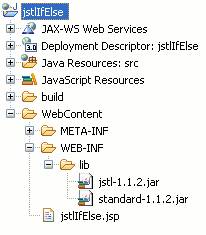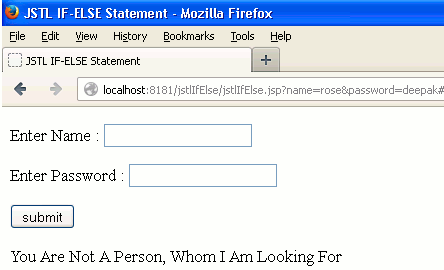Ads

Tutorials

# IF-ELSE In JSTL

IF-ELSE in JSTL section explains you how the if-else tag can be use in web page development.

IF-ELSE in JSTL section explains you how the if-else tag can be use in web page development.

## IF-ELSE In JSTL

In this section we will read about implementation of if-else tag in JSTL. We will see the implementation of this tag using a simple example.

In JSTL if-else tag is not implemented directly like the if-else statement is used on the Java/JSP coding. Implementation of if-else tag in JSTL coding is not same as Java/JSP coding. To implement the if-else in JSTL coding there are some tags defined in JSTL these are as follows :

<c:choose> : This tag is like the 'if' notion used in Java/JSP programming. This is a simple tag that is used to perform the conditional operations using the tags <when> and <otherwise>.

<c:when> : Sub tag of <c:choose> tag. It is used to provide a condition to be evaluate. The condition should be evaluated to boolean expression. If this boolean expression evaluates to true then the rest of the body of this tag is evaluated.

<c:otherwise> : Sub tag of <c:choose> tag. It is used with the <c:when> tag. Body of this tag is evaluated when the condition provided to the <c:when> tag is evaluated to 'false'.

Combination of above three tags are used as an if-else statement in JSTL programming. Sample code given below expresses you how this tag is used.:

```<c:choose>
<c:when test="\${condition1}">
...
</c:when>
<c:when test="\${condition2}">
...
</c:when>
<c:otherwise>
...
</c:otherwise>
</c:choose>

```

Example

Here an example is being given which will demonstrate you how to use the if-else conditional statement in JSTL. In this example we will create a simple JSP page where we will use the JSTL <c:choose>, <c:when> and <c:otherwise> tag to apply the if-else operation. We will use the Eclipse IDE and Tomcat 7 server for writing and deploying the application. At first we will create a new dynamic web project then we will create a JSP page inside the Web Content folder then we will use the above mentioned tag in the JSP page.

Directory StructurejstlIfElse.jsp

```<%@ page language="java" contentType="text/html; charset=ISO-8859-1"
pageEncoding="ISO-8859-1"%>
<!DOCTYPE html PUBLIC "-//W3C//DTD HTML 4.01 Transitional//EN" "http://www.w3.org/TR/html4/loose.dtd">
<%@ taglib uri="http://java.sun.com/jsp/jstl/core" prefix="c" %>
<html>
<head>
<meta http-equiv="Content-Type" content="text/html; charset=ISO-8859-1">
<title>JSTL IF-ELSE Statement</title>
</head>
<body>
<form action="#">
<p>Enter Name :
<input type="text" name="name"/></p>
<p>Enter Password :
<input type="password" name="password"/></p>
<p><input type="submit" value="submit"/></p>
</form>
<%
String name = request.getParameter("name");
String password = request.getParameter("password");
%>
<%
if(!(name == null || (name.equals(""))))
{
%>
<c:set var="nm" value="<%=name %>"/>
<c:set var="psw" value="<%=password %>"/>
<c:choose>
<c:when test="\${(nm == 'deepak') && (psw == 'deepak')}">
<p>Welcome, <c:out value="\${nm }"/></p>
</c:when>
<c:otherwise>
<p>You Are Not A Person, Whom I Am Looking For</p>
</c:otherwise>
</c:choose>
<%
}
%>
</body>
</html>

```

Output

When you will deploy the above example you will get the output as follows :When you will input the value of fields 'name' and 'password' to 'deepak' and 'deepak' then the body of <c:when> tag will be evaluated and the output will be as follows :When you will input the value of fields 'name' and 'password' other than 'deepak' then the body of <c:otherwise> tag will be evaluated and then the output will be as follows :Download Source Code

Ads

Ads